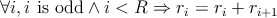# G :: Khayyam - Pascal’s Triangle

Time Limit: 1 Second    Memory Limit: 65536 KB

“Pascal’s triangle is a triangular array of the binomial coefficients. The rows of Pascal’s triangle are conventionally enumerated starting with row n = 1 at the top. The entries in each row are numbered from the left beginning with k = 1. A simple construction of the triangle proceeds in the following manner. On row 1, write only the number 1. Then, to construct the elements of following rows, add the number above and to the left with the number above and to the right to find the new value. If either the number to the right or left is not present, substitute a zero in its place. For example, the first number in the first row is 1 (the sum of 0 and 1), whereas the numbers 1 and 3 in the fourth row are added to produce the number 4 in the fifth row.” –WikipediaWe choose an arbitrary row like R that its elements are {r1,...,rR} and we change this row in the following manner:Now we want to know what happens to the Mth entry in the Nth row.
For example, if we choose the 2nd row, then its elements change to {2, 1} and then the 2nd entry in the 4th row would be 5.

## Input

The input contains several test cases.
In the first line of input comes T (0 < T < 64), the number of test cases.
Each test case consists of two lines. The first line contains an integer R (1 ≤ R ≤ 50000), the index of the row that we are going to change as described above.
The second line contains integers N and M (R < N < 200000, 1 ≤ M ≤ N).

## Output

For each test case, output the Mth element of the Nth row of the triangle, modulo 1,000,000,009 (109+9 is a prime number).

```2
5
7 5
7
9 5```

## Sample Output

```19
96```
Submit

Source: 12th Iran Nationwide Internet Contest - Final